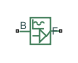# Sinusoidal Translational Velocity Source

Produce sinusoidal translational velocity

•Libraries:
Simscape / Driveline / Sources

## Description

The Sinusoidal Translational Velocity Source block produces sinusoidal translational velocity with a constant offset.

The relative translational velocity between the base and follower interfaces is

`${v}_{FB}={v}_{F}-{v}_{B},$`

where:

• v FB is the relative translational velocity.

• vF is the absolute translational velocity at the follower interface.

• vB is the absolute translational velocity at the base interface.

The defining equation for the sinusoidal translational velocity that the block generates is

`$v={v}_{o}+A*\mathrm{sin}\left(2\pi ft+\phi \right),$`

where:

• v is the output translational velocity.

• vo is the offset translational velocity of the output.

• A is the peak amplitude of the sinusoid.

• f is frequency of the sinusoid in Hz.

• t is time.

• φ is phase shift of the sinusoid.

## Ports

### Conserving

expand all

Mechanical translational conserving port associated with the base interface.

Mechanical translational conserving port associated with the follower interface.

## Parameters

expand all

Average value that the output sinusoidal translational velocity oscillates about.

Peak amplitude of the output sinusoidal translational velocity.

Phase shift of the output sinusoidal translational velocity

Frequency of the output sinusoidal translational velocity. The frequency must be greater than or equal to 0.

## Version History

Introduced in R2016b